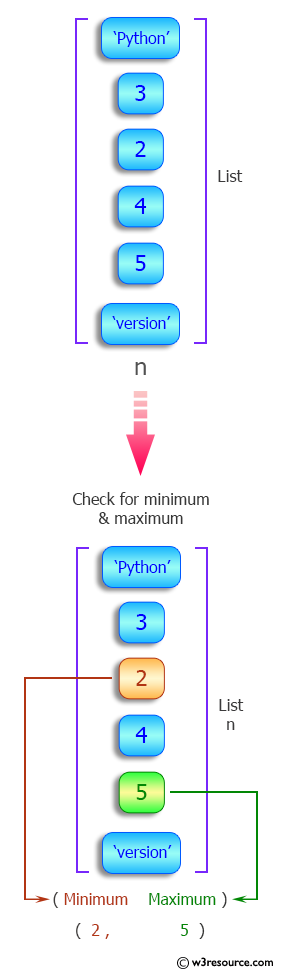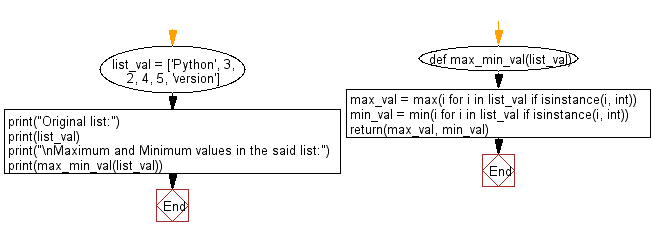﻿ Python: Find the maximum and minimum values in a given heterogeneous list - w3resource# Python: Find the maximum and minimum values in a given heterogeneous list

## Python List: Exercise - 99 with Solution

Write a Python program to find the maximum and minimum values in a given heterogeneous list.

Sample Solution:

Python Code:

``````def max_min_val(list_val):
max_val = max(i for i in list_val if isinstance(i, int))
min_val = min(i for i in list_val if isinstance(i, int))
return(max_val, min_val)

list_val = ['Python', 3, 2, 4, 5, 'version']
print("Original list:")
print(list_val)
print("\nMaximum and Minimum values in the said list:")
print(max_min_val(list_val))
```
```

Sample Output:

```Original list:
['Python', 3, 2, 4, 5, 'version']

Maximum and Minimum values in the said list:
(5, 2)
```

Pictorial Presentation:Flowchart:## Visualize Python code execution:

The following tool visualize what the computer is doing step-by-step as it executes the said program:

Python Code Editor:

Have another way to solve this solution? Contribute your code (and comments) through Disqus.

What is the difficulty level of this exercise?

Test your Python skills with w3resource's quiz

﻿

## Python: Tips of the Day

```print(2_000_000)
```2000000